# Beginners Machine Learning Explained Simply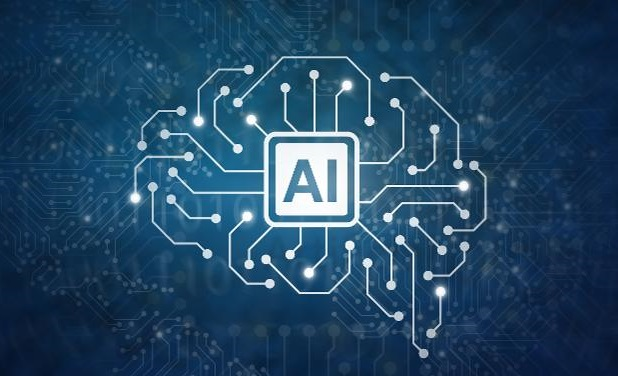It is predicted that 80% of emerging technologies will have AI foundation by 2021

AI has its applications everywhere. Auto driving, face recognition, language translation, market prediction, chatbots, text to speech, ads and movies suggestion, application in medical science, game bots, and many more.

Do you want to know how these applications work?

Do you want to make these yourself?

Artificial intelligence is a continuously growing field.

Till now, AI can already :

• Read : and summarize a long text for you. Like it does in google search engine.
• Write : Can make jokes and write a poem. Even a novel has been generated by AI that was short-listed for an award.
• See : Auto driving cars, facial recognition.
• Hear and understand : Virtual assistants. In some applications, even it can alert you if it hears a gunshot.
• Play Games : DeepMind’s AlphaZero has already beat the World Chess Champion. And do you know how much time it took to learn chess completely from scratch ? Just 4 hours.
• It can Speak, Smell, touch, Move, Create, debate and …..

Despite all these, we can say that we are at the beginning of the development of AI. Like here, shown in the picture.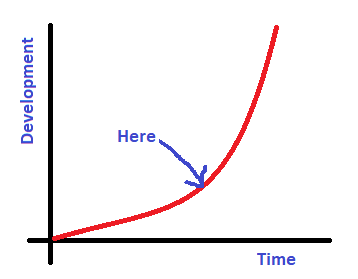In this article, my purpose is to grow a spark inside you towards artificial intelligence and machine learning. So that if you like, you can get your hands on to this amazing growing field !

• know what is AI and ML
• know similarity between AI and human brain
• Make a simple model to predict house prices, using a simple but widely used model.
• And at last, find some courses that you can take to learn Machine Learning from beginner to advance.

Note : The model is implemented in python. But if you don’t know python, you need not to worry. You can just learn the model for a start and implement later.

So, are you excited ?

Let’s get started !

### What is Artificial Intelligence ?

In one sentence, it is “An ability of a computer to mimic human”. It’s that simple.

An ability of a computer to mimic human

And Machine Learning is an application used in AI, which uses models to help computers learn itself from the data. Computer takes data, recognizes patterns from it and learns from the data.

This can be well understood by the example below :

#### Human Brain and AI

Suppose you are a kid and your parents shows you something which you don’t know what is. And they say, “It is X”. And some days later, they show you that again, and they say, “Hey dear, it’s X”.

Soon after a certain number of times your parents show you the thing with the label X, you can classify that as X, even if the thing X is of slighly different in kind.

In the above example, your brain got some data, it recognized patterns from it, attached a label X to it and now it knows what it is. That is how human brain works. But its not common for computers. Computer can’t know what it is, if that is something it has never seen.

But with the Machine Learning model, if you show similar objects with the label, for many times, it can recognize the similar new object next time it sees it. Like if, you show your face telling it that it is “you”, then the next time it will see you, it will recognize you, even if you are wearing glasses or are different

### The reason for high rise in Machine Learning ?

Machine Learning has been used since the 1950s or before. But the 2 reasons why it has gain much development are the following :

1. Internet and Data : The rate at which we are producing data everyday is the highest till now.
2. Computational Power : The computational power is increasing every year. This increase allowed us to train bigger data faster.

So that was some info regarding AI and ML. Now you will learn to make a simple but effective ML model.

We will make a model which predicts house price. The data used here has house sq. foot area and their orginal price. After training the model, it will be able to predict price for new houses.

Sq. Foor AreaPrice
1500158900
1700169850
1750178950
1900?? to predict
1850?? to predict

The data on graph looks like below. If we can draw an approximate line that fits the data, we can predict price of any house.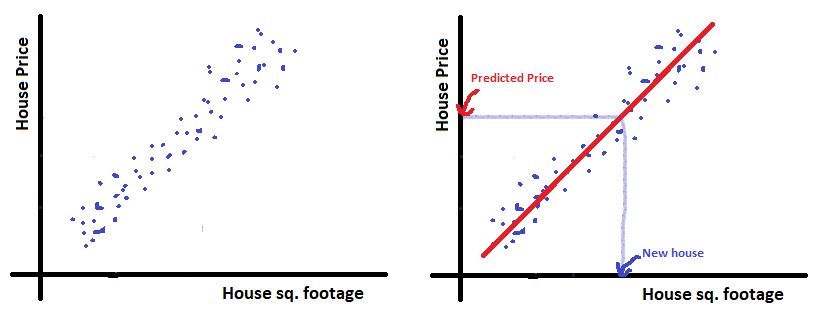Now we will discuss the model to get that approximate line that fits the data.

### Model

So, how can you draw this line?

The line which will fit our data, will have the least sum of perpendicular distance from all the points to the line.

Thus the approximate line can be drawn by taking the sum of all the perpendicular distances from all the points to a random line and making that sum least possible

To get the line, we need first parameters for the line.

Let say it is represented by a Theta array.

theta = [ [a], [b] ]

And thus the line will be Y = aX + b, with X as sq.ft area and Y as price.

and our data is :

data = [ [X1, Y1], [X2, Y2], […, …] ]

We will seperate data X and predictions Y. And then add column vector of 1’s to X.

X = [ [X1, 1], [X2, 1], […, …] ]

Y = [ [Y1], [Y2], […] ]

So that, now matrix multiplication of Theta and X will give us YNow,

#### Step 1 : Cost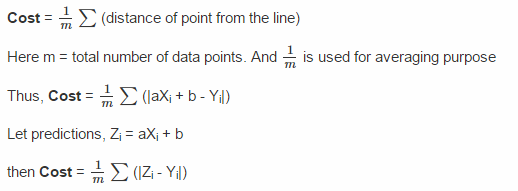#### Step 2 : Minimize Cost

The value of cost depend on the parameter vector Theta.

We will initialize Theta with [, ], which will make the cost value largest.

How do you think we can minimize cost ?

How about finding local minima ?

To find the local minima, we will differentiate cost w.r.t theta. This will provide us with slope.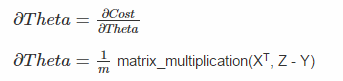How did the above equation came ? Find differentiation of Cost wrt Theta. Hint : First differentiate cost with theta, then multiply the differentiation of (Z – Y) with theta. The transpose of X is taken to match the matrix multiplication

Update Theta as : Theta = Theta – alpha x dTheta

Here, alpha is some costant number.

By performing the above 2 steps from many number of time, we will reach the local minima of cost.

##### How ?
1. Suppose the slope i.e, d_theta is positive, then theta value will decrease and thus the value of cost with decrease.
2. Suppose the slope is negative, then theta value will increase and cost value will decreases, and thus leading the cost closer to local minima.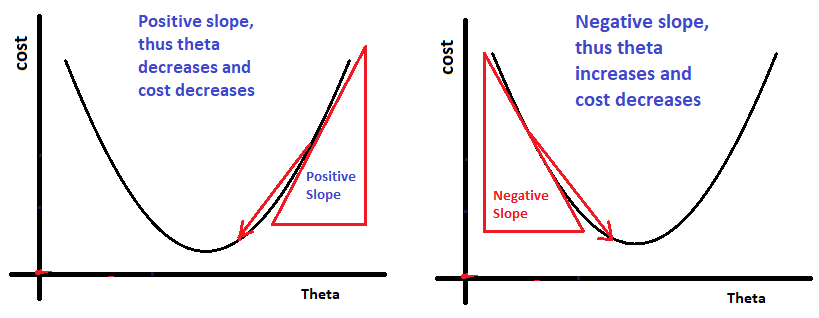If we perform the above 2 steps for many times, let say ‘iteration’ number of times, we will reach the local minima.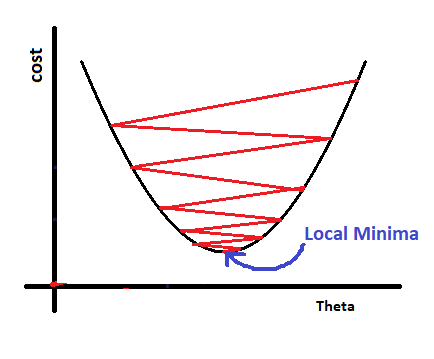The implementation is like this :

```loop from 1 to iterations times:
# This will give us predictions
Z = matrix_mulitplication(X, Theta)
Cost = (1/m)*sum( abs(Z - Y) )
# This update will take us closer to its local minma
d_Theta = (1/m)*matrix_multiplication(X.T, Z-Y)
Theta = Theta - alpha*d_theta```

If you find the above two steps a bit difficult to understand, don’t worry, as you will implement it yourself, you will get more clear. I recommend you to think thoroughly while implementing.

So, you have seen how our model looks like and how to build it.

Let us now code our model.

You will see how short it is, but does some great things.

### Full Implementation in Python :

I have used ‘ipython jupyter notebook’ to write the code, and I recommend you the same for every machin learning models

The dataset I have used to train the model, is available here : Dataset

```import numpy as np
import matplotlib.pyplot as plt
data = np.loadtxt('data.txt', delimiter = ',', dtype = int)
X = data[:, :1]
Y = data[:, 1].reshape(X.shape, 1)
X = np.hstack((X, np.ones((X.shape,1)) ))```
```# Visualization of Dataset
plt.scatter(X[:, 0], Y)
plt.show()```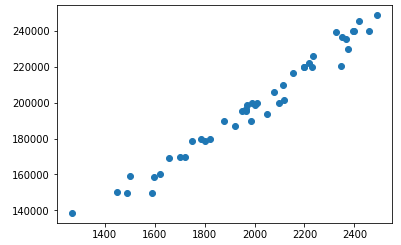```def model(X, Y, alpha, iterations):
cost_list = []
m = Y.shape
theta = np.zeros((X.shape,1))
for i in range(iterations+1):
A = np.dot(X, theta)
cost = (1/m)*np.sum(np.abs(A - Y))
d_theta = (1/m)*np.dot(X.T, A-Y)
theta = theta - alpha*d_theta
if(i % (iterations/10) == 0):
print("cost after", i, "iterations is :", cost)
cost_list.append(cost)
return theta, np.array(cost_list)
theta, cost_list = model(X, Y, alpha = 0.00000005, iterations = 50)```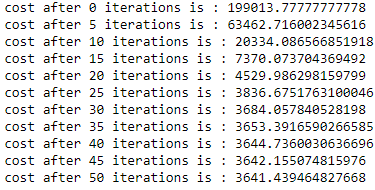Do you see, how the value of cost is decreasing and then almost remains cost. This shows that it has reached local minima.

Our mode is trained ! Lets us see, how it is predicting !

```new_houses = np.array([[1547, 1], [1896, 1], [1934, 1], [2800, 1], [3400, 1], [5000, 1]])
for house in new_houses :
print("Our model predicts the price of house with", house, "sq. ft. area as : \$", round(np.dot(house, theta), 2))```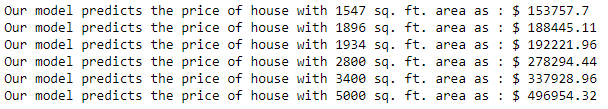#### Congratulations, you have implemented your first Machine Learning model!

This was a good implementation as beginner. You did very nice!

Here we used only 1 feature of data as just our house sq.ft. area. The real-world application uses many more number of features. And that can be, garage area, bathroom area, total number of rooms, locality, furniture quality, etc.

If the number of features increases, the only thing you need to change is parameter Theta. If the total number of features are N, then take the size of theta as (N+1, 1) vector, and everything else remains the same. In our case, N was 1 i.e, house sq. ft. area.

The model you just implemented is very famous model, which machine learning engineers uses all the time. And its name is SVM (Support Vector Machine). When you will take an actual machine learning course, then you will be more familiar with the terms.

There are many other models as well in Machine Learning, and the implementation of different models depends on the application we are trying to build and on dataset.

The most famous of them are Neural Networks.

### Courses you can take to become a Machine Learning expert :

1. Deep Learning Specialization on Coursera – by Andrew Ng. This specialization teaches you Neural Networks. The projects in it are very awesome like, image recognition, language translation(Spanish to English), Emojifying the text. I am sure you will love doing it yourself.This is more suitable if you are interested in making AI applications yourself.